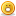# Thread: diff between parameter passing by reference and passing by value

1. ## diff between parameter passing by reference and passing by value

wat is difference between parameter passing by reference and parameter passing by value? and example?Reply With Quote

2. ## Re: diff between parameter passing by reference and passing by value

Parameters passing by reference means we are directly passing the memory address where value is saved.

Parameters passing by value means we are passing the parameter value only.

check this link for a better example.
http://www.cs.princeton.edu/~lworthi...s_val_ref.htmlReply With Quote

3. ## Re: diff between parameter passing by reference and passing by value

Parameter passing by value means only a value is passed to the function and any changes to the parameters doesnt change the original arguments passed to the function.
Eg

main()
{
int a=10,b=20,c=0;
c=func(a,b); // pass by value
print a // prints 10
print b // prints 20
};

func(int x,int y) // recieves values 10 & 20
{
x=x+1; // adds 1 to x
y=y+1; // adds 1 to y
print x; // prints 11
print y; // ptints 21
}

Parameter passing by reference means the address of the original arguments are passed to the function and any changes to the parameters change the original arguments also.
Eg

main()
{
int a=10,b=20,c=0;
c=func(&a,&b); // pass by reference
print a // prints 11
print b // ptints 21};

func(int *x,int *y) // recieves references to a & b
{
*x=*x+1; // adds 1 to a
*y=*y+1; // adds 1 to b
print *x; // prints a as 11
print *y; // prints b as 21
}Reply With Quote

4. ## Re: diff between parameter passing by reference and passing by value

Excellent example !!! Its good one :-)Reply With Quote

5.

####Posting Permissions

• You may not post new threads
• You may not post replies
• You may not post attachments
• You may not edit your posts
•